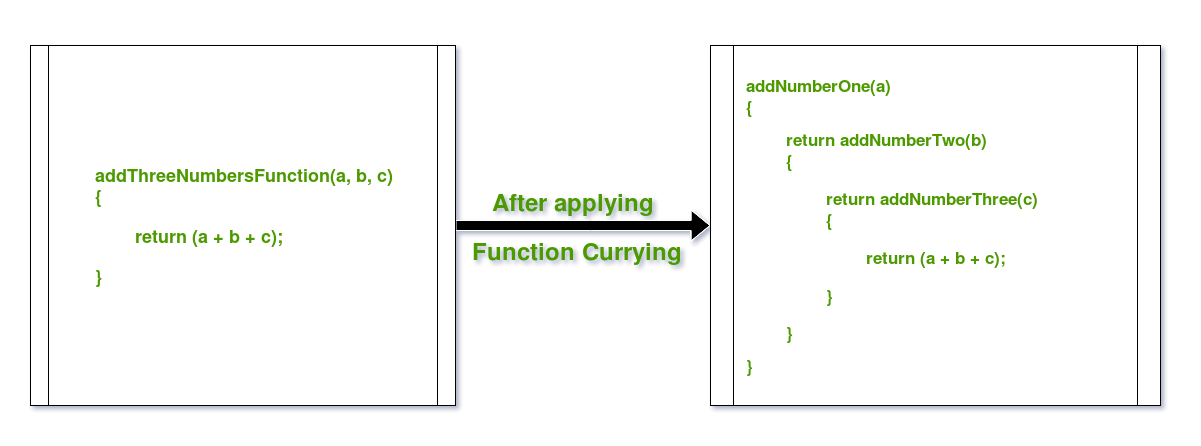# Currying Functions in Java with Examples

Function Currying is a concept of breaking a function with many arguments into many functions with single argument in such a way, that the output is same. In other words, its a technique of simplifying a multi-valued argument function into single-valued argument multi-functions.

Consider the example to clear the concept:Currying breaks down higher order functions into a series of smaller cascaded functions which take in one argument and return a function except for the last cascaded function which returns the desired value.

For example:

Let there be a function which maps asCurrying the above function will produceThusmaps fromto a function which in turn maps fromtoThe above mathematical expression can also be represented as:Hence,Below are some examples in Java to demonstrate Function Currying:

Example 1: Adding 2 numbers using Function Currying

 `// Java Program to demonstrate Function Currying ` ` `  `import` `java.util.function.Function; ` ` `  `public` `class` `GFG { ` `    ``public` `static` `void` `main(String args[]) ` `    ``{ ` ` `  `        ``// Using Java 8 Functions ` `        ``// to create lambda expressions for functions ` `        ``// and with this, applying Function Currying ` ` `  `        ``// Curried Function for Adding u & v ` `        ``Function > ` `            ``curryAdder = u -> v -> u + v; ` ` `  `        ``// Calling the curried functions ` ` `  `        ``// Calling Curried Function for Adding u & v ` `        ``System.out.println(``"Add 2, 3 :"` `                           ``+ curryAdder ` `                                 ``.apply(``2``) ` `                                 ``.apply(``3``)); ` ` `  `        ``} ` `} `

Output:

```Add 2, 3 :5
```

Example 2: Multiplying 2 numbers using Function Currying

 `// Java Program to demonstrate Function Currying ` ` `  `import` `java.util.function.Function; ` ` `  `public` `class` `GFG { ` `    ``public` `static` `void` `main(String args[]) ` `    ``{ ` ` `  `        ``// Using Java 8 Functions ` `        ``// to create lambda expressions for functions ` `        ``// and with this, applying Function Currying ` ` `  `        ``// Curried Function for Multiplying u & v ` `        ``Function > ` `            ``curryMulti = u -> v -> u * v; ` ` `  `        ``// Calling the curried functions ` `        `  `        ``// Calling Curried Function for Multiplying u & v ` `        ``System.out.println(``"Multiply 2, 3 :"` `                           ``+ curryMulti ` `                                 ``.apply(``2``) ` `                                 ``.apply(``3``)); ` `    ``} ` `} `

Output:

```Multiply 2, 3 :6
```

Example 3: Adding 3 numbers using Function Currying

 `// Java Program to demonstrate Function Currying ` ` `  `import` `java.util.function.Function; ` ` `  `public` `class` `GFG { ` `    ``public` `static` `void` `main(String args[]) ` `    ``{ ` ` `  `        ``// Using Java 8 Functions ` `        ``// to create lambda expressions for functions ` `        ``// and with this, applying Function Currying ` ` `  `        ``// Curried Function for Adding u, v & w ` `        ``Function > > ` `            ``triadder = u -> w -> v -> u + w + v; ` ` `  `        ``// Calling the curried functions ` ` `  `        ``// Calling Curried Function for Adding u, v & w ` `        ``System.out.println(``"Add 2, 3, 4 :"` `                           ``+ triadder ` `                                 ``.apply(``2``) ` `                                 ``.apply(``3``) ` `                                 ``.apply(``4``)); ` `    ``} ` `} `

Output:

```Add 2, 3, 4 :9
```

Don’t stop now and take your learning to the next level. Learn all the important concepts of Data Structures and Algorithms with the help of the most trusted course: DSA Self Paced. Become industry ready at a student-friendly price.

My Personal Notes arrow_drop_upCheck out this Author's contributed articles.

If you like GeeksforGeeks and would like to contribute, you can also write an article using contribute.geeksforgeeks.org or mail your article to contribute@geeksforgeeks.org. See your article appearing on the GeeksforGeeks main page and help other Geeks.

Please Improve this article if you find anything incorrect by clicking on the "Improve Article" button below.

Article Tags :
Practice Tags :

8

Please write to us at contribute@geeksforgeeks.org to report any issue with the above content.